Anúncio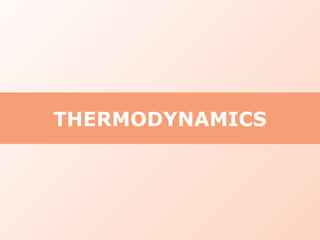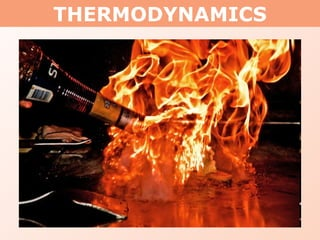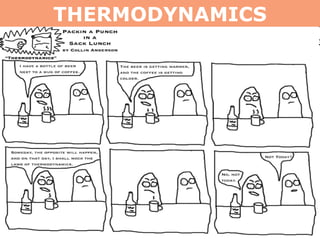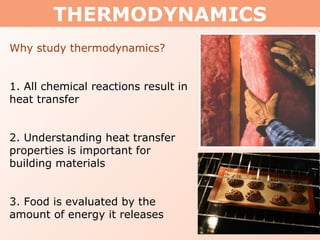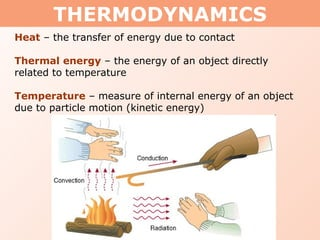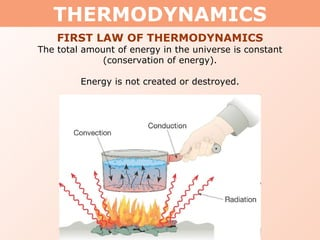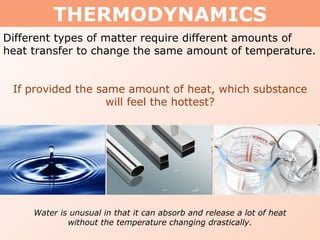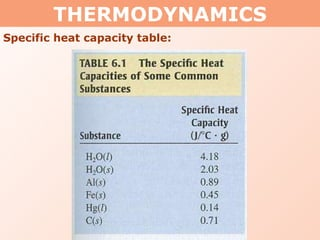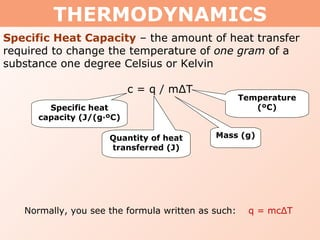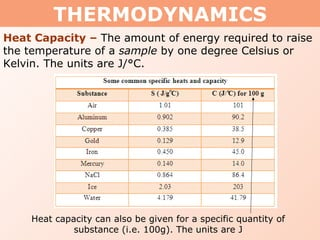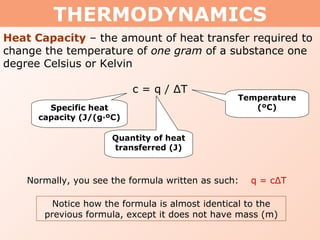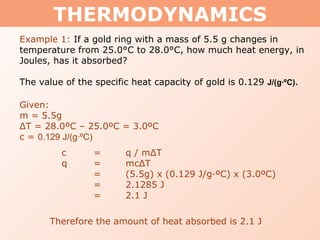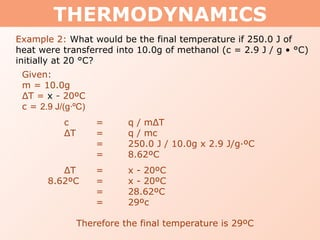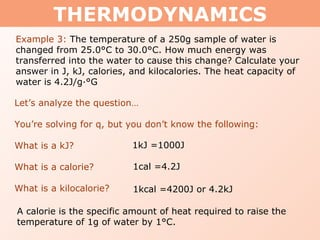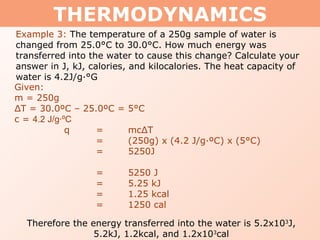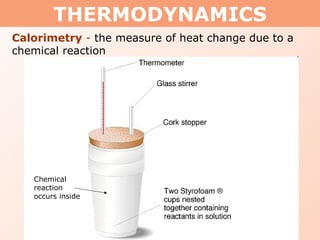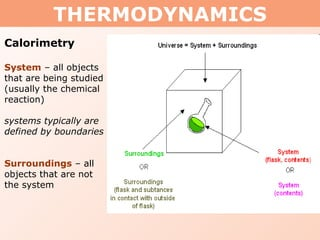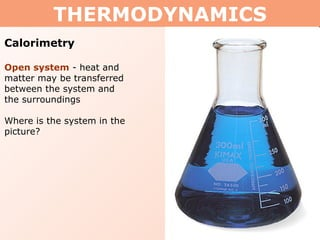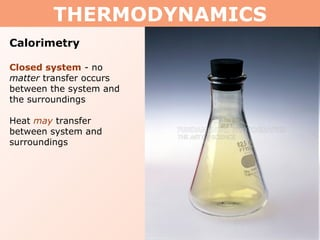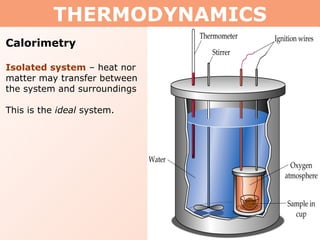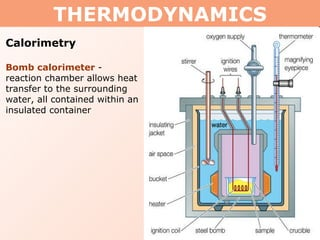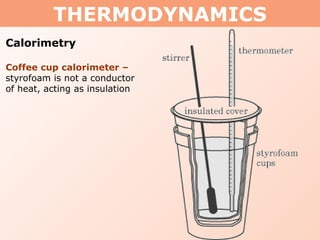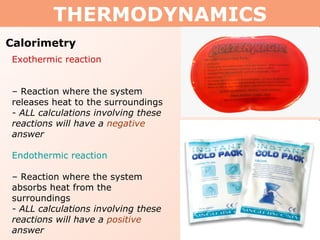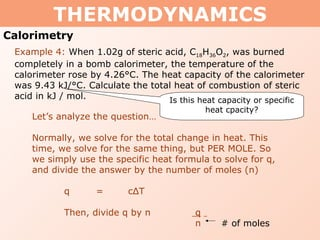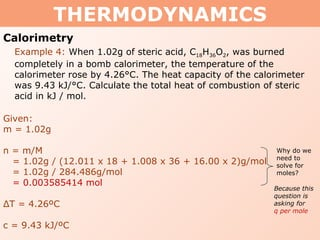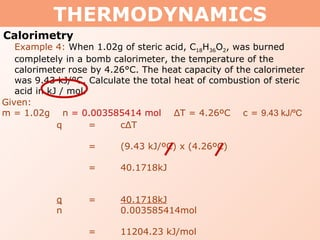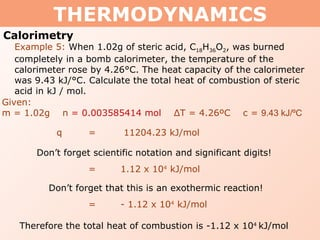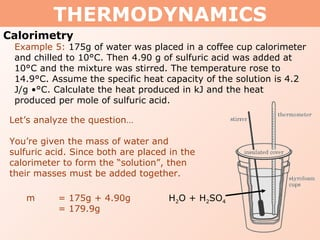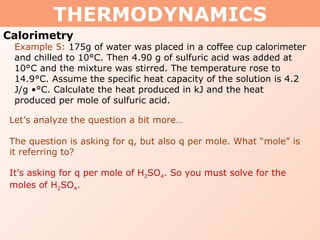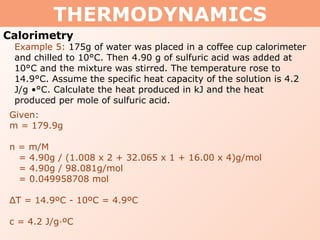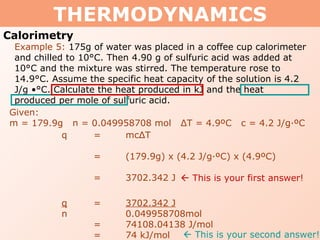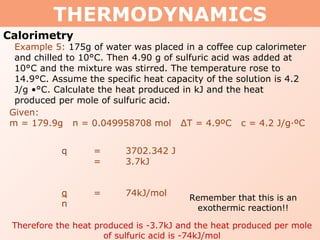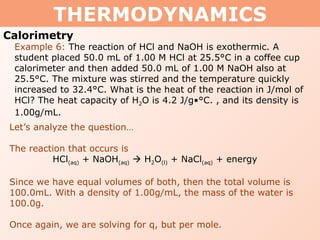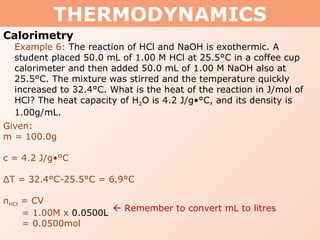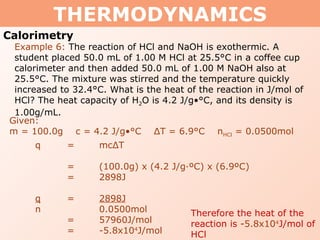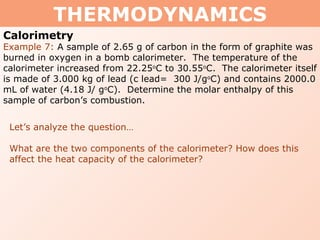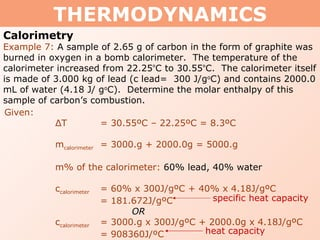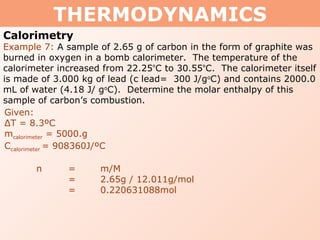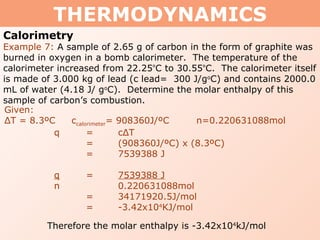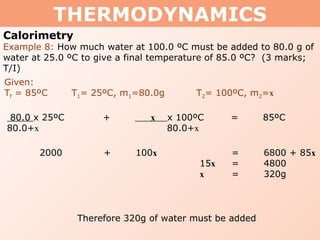1 de 40
Anúncio

### Tang 01 heat capacity and calorimetry

1. THERMODYNAMICS
2. THERMODYNAMICS
3. THERMODYNAMICS
4. THERMODYNAMICS Why study thermodynamics? 1. All chemical reactions result in heat transfer 2. Understanding heat transfer properties is important for building materials 3. Food is evaluated by the amount of energy it releases
5. THERMODYNAMICS Heat – the transfer of energy due to contact Thermal energy – the energy of an object directly related to temperature Temperature – measure of internal energy of an object due to particle motion (kinetic energy)
6. THERMODYNAMICS FIRST LAW OF THERMODYNAMICS The total amount of energy in the universe is constant (conservation of energy). Energy is not created or destroyed.
7. THERMODYNAMICS Different types of matter require different amounts of heat transfer to change the same amount of temperature. If provided the same amount of heat, which substance will feel the hottest? Water is unusual in that it can absorb and release a lot of heat without the temperature changing drastically.
8. THERMODYNAMICS Specific heat capacity table:
9. THERMODYNAMICS Specific Heat Capacity – the amount of heat transfer required to change the temperature of one gram of a substance one degree Celsius or Kelvin c = q / m Δ T Specific heat capacity (J/(g·ºC) Quantity of heat transferred (J) Mass (g) Temperature (ºC) Normally, you see the formula written as such: q = mc Δ T
10. THERMODYNAMICS Heat Capacity – The amount of energy required to raise the temperature of a sample by one degree Celsius or Kelvin. The units are J/°C. Heat capacity can also be given for a specific quantity of substance (i.e. 100g). The units are J
11. THERMODYNAMICS Heat Capacity – the amount of heat transfer required to change the temperature of one gram of a substance one degree Celsius or Kelvin c = q / Δ T Specific heat capacity (J/(g·ºC) Quantity of heat transferred (J) Temperature (ºC) Normally, you see the formula written as such: q = c Δ T Notice how the formula is almost identical to the previous formula, except it does not have mass (m)
12. THERMODYNAMICS Example 1: If a gold ring with a mass of 5.5 g changes in temperature from 25.0°C to 28.0°C, how much heat energy, in Joules, has it absorbed? The value of the specific heat capacity of gold is 0.129 J/(g·ºC) . Given: m = 5.5g Δ T = 28.0ºC – 25.0ºC = 3.0ºC c = 0.129 J/(g·ºC) c = q / m Δ T q = mc Δ T = (5.5g) x (0.129 J/g·ºC) x (3.0ºC) = 2.1285 J = 2.1 J Therefore the amount of heat absorbed is 2.1 J
13. THERMODYNAMICS Example 2: What would be the final temperature if 250.0 J of heat were transferred into 10.0g of methanol (c = 2.9 J / g • °C) initially at 20 °C? Given: m = 10.0g Δ T = x - 20ºC c = 2.9 J/(g·ºC) c = q / m Δ T Δ T = q / mc = 250.0 J / 10.0g x 2.9 J/g·ºC = 8.62 ºC Therefore the final temperature is 29ºC Δ T = x - 20 ºC 8.62ºC = x - 20ºC = 28.62ºC = 29ºc
14. THERMODYNAMICS Example 3: The temperature of a 250g sample of water is changed from 25.0°C to 30.0°C. How much energy was transferred into the water to cause this change? Calculate your answer in J, kJ, calories, and kilocalories. The heat capacity of water is 4.2J/g∙°G Let’s analyze the question… You’re solving for q, but you don’t know the following: What is a kJ? What is a calorie? What is a kilocalorie? 1kJ =1000J 1cal =4.2J 1kcal =4200J or 4.2kJ A calorie is the specific amount of heat required to raise the temperature of 1g of water by 1°C.
15. THERMODYNAMICS Example 3: The temperature of a 250g sample of water is changed from 25.0°C to 30.0°C. How much energy was transferred into the water to cause this change? Calculate your answer in J, kJ, calories, and kilocalories. The heat capacity of water is 4.2J/g∙°G Given: m = 250g Δ T = 30.0 ºC – 25.0ºC = 5°C c = 4.2 J/g·ºC q = mc Δ T = (250g) x (4.2 J/g·ºC) x (5°C) = 5250J = 5250 J = 5.25 kJ = 1.25 kcal = 1250 cal Therefore the energy transferred into the water is 5.2x10 3 J, 5.2kJ, 1.2kcal, and 1.2x10 3 cal
16. THERMODYNAMICS Calorimetry - the measure of heat change due to a chemical reaction Chemical reaction occurs inside
17. THERMODYNAMICS Calorimetry System – all objects that are being studied (usually the chemical reaction) systems typically are defined by boundaries Surroundings – all objects that are not the system
18. THERMODYNAMICS Calorimetry Open system - heat and matter may be transferred between the system and the surroundings Where is the system in the picture?
19. THERMODYNAMICS Calorimetry Closed system - no matter transfer occurs between the system and the surroundings Heat may transfer between system and surroundings
20. THERMODYNAMICS Calorimetry Isolated system – heat nor matter may transfer between the system and surroundings This is the ideal system.
21. THERMODYNAMICS Calorimetry Bomb calorimeter - reaction chamber allows heat transfer to the surrounding water, all contained within an insulated container
22. THERMODYNAMICS Calorimetry Coffee cup calorimeter – styrofoam is not a conductor of heat, acting as insulation
23. THERMODYNAMICS Calorimetry Exothermic reaction – Reaction where the system releases heat to the surroundings - ALL calculations involving these reactions will have a negative answer Endothermic reaction – Reaction where the system absorbs heat from the surroundings - ALL calculations involving these reactions will have a positive answer
24. THERMODYNAMICS Calorimetry Let’s analyze the question… Normally, we solve for the total change in heat. This time, we solve for the same thing, but PER MOLE. So we simply use the specific heat formula to solve for q, and divide the answer by the number of moles (n) q = c Δ T Then, divide q by n q n Example 4: When 1.02g of steric acid, C 18 H 36 O 2 , was burned completely in a bomb calorimeter, the temperature of the calorimeter rose by 4.26°C. The heat capacity of the calorimeter was 9.43 kJ/°C. Calculate the total heat of combustion of steric acid in kJ / mol. Is this heat capacity or specific heat cpacity? # of moles
25. THERMODYNAMICS Calorimetry Example 4: When 1.02g of steric acid, C 18 H 36 O 2 , was burned completely in a bomb calorimeter, the temperature of the calorimeter rose by 4.26°C. The heat capacity of the calorimeter was 9.43 kJ/°C. Calculate the total heat of combustion of steric acid in kJ / mol. Given: m = 1.02g n = m/M = 1.02g / (12.011 x 18 + 1.008 x 36 + 16.00 x 2)g/mol = 1.02g / 284.486g/mol = 0.003585414 mol Δ T = 4.26ºC c = 9.43 kJ/ºC Because this question is asking for q per mole Why do we need to solve for moles?
26. THERMODYNAMICS Calorimetry Given: m = 1.02g n = 0.003585414 mol Δ T = 4.26ºC c = 9.43 kJ/ºC q = c Δ T = (9.43 kJ/ºC) x (4.26ºC) = 40.1718kJ q = 40.1718kJ n 0.003585414mol = 11204.23 kJ/mol Example 4: When 1.02g of steric acid, C 18 H 36 O 2 , was burned completely in a bomb calorimeter, the temperature of the calorimeter rose by 4.26°C. The heat capacity of the calorimeter was 9.43 kJ/°C. Calculate the total heat of combustion of steric acid in kJ / mol.
27. THERMODYNAMICS Calorimetry Given: m = 1.02g n = 0.003585414 mol Δ T = 4.26ºC c = 9.43 kJ/ºC q = 11204.23 kJ/mol Example 5: When 1.02g of steric acid, C 18 H 36 O 2 , was burned completely in a bomb calorimeter, the temperature of the calorimeter rose by 4.26°C. The heat capacity of the calorimeter was 9.43 kJ/°C. Calculate the total heat of combustion of steric acid in kJ / mol. Don’t forget scientific notation and significant digits! = 1.12 x 10 4 kJ/mol Therefore the total heat of combustion is -1.12 x 10 4 kJ/mol Don’t forget that this is an exothermic reaction! = - 1.12 x 10 4 kJ/mol
28. THERMODYNAMICS Calorimetry Example 5: 175g of water was placed in a coffee cup calorimeter and chilled to 10°C. Then 4.90 g of sulfuric acid was added at 10°C and the mixture was stirred. The temperature rose to 14.9°C. Assume the specific heat capacity of the solution is 4.2 J/g •°C. Calculate the heat produced in kJ and the heat produced per mole of sulfuric acid. Let’s analyze the question… You’re given the mass of water and sulfuric acid. Since both are placed in the calorimeter to form the “solution”, then their masses must be added together. m = 175g + 4.90g = 179.9g H 2 O + H 2 SO 4
29. THERMODYNAMICS Calorimetry Example 5: 175g of water was placed in a coffee cup calorimeter and chilled to 10°C. Then 4.90 g of sulfuric acid was added at 10°C and the mixture was stirred. The temperature rose to 14.9°C. Assume the specific heat capacity of the solution is 4.2 J/g •°C. Calculate the heat produced in kJ and the heat produced per mole of sulfuric acid. Let’s analyze the question a bit more… The question is asking for q, but also q per mole. What “mole” is it referring to? It’s asking for q per mole of H 2 SO 4 . So you must solve for the moles of H 2 SO 4 .
30. THERMODYNAMICS Calorimetry Example 5: 175g of water was placed in a coffee cup calorimeter and chilled to 10°C. Then 4.90 g of sulfuric acid was added at 10°C and the mixture was stirred. The temperature rose to 14.9°C. Assume the specific heat capacity of the solution is 4.2 J/g •°C. Calculate the heat produced in kJ and the heat produced per mole of sulfuric acid. Given: m = 179.9g n = m/M = 4.90g / (1.008 x 2 + 32.065 x 1 + 16.00 x 4)g/mol = 4.90g / 98.081g/mol = 0.049958708 mol Δ T = 14.9ºC - 10ºC = 4.9ºC c = 4.2 J/g·ºC
31. THERMODYNAMICS Calorimetry Example 5: 175g of water was placed in a coffee cup calorimeter and chilled to 10°C. Then 4.90 g of sulfuric acid was added at 10°C and the mixture was stirred. The temperature rose to 14.9°C. Assume the specific heat capacity of the solution is 4.2 J/g •°C. Calculate the heat produced in kJ and the heat produced per mole of sulfuric acid. Given: m = 179.9g n = 0.049958708 mol Δ T = 4.9ºC c = 4.2 J/g·ºC q = mc Δ T = (179.9g) x (4.2 J/g·ºC) x (4.9ºC) = 3702.342 J q = 3702.342 J n 0.049958708mol = 74108.04138 J/mol = 74 kJ/mol  This is your first answer!  This is your second answer!
32. THERMODYNAMICS Calorimetry Example 5: 175g of water was placed in a coffee cup calorimeter and chilled to 10°C. Then 4.90 g of sulfuric acid was added at 10°C and the mixture was stirred. The temperature rose to 14.9°C. Assume the specific heat capacity of the solution is 4.2 J/g •°C. Calculate the heat produced in kJ and the heat produced per mole of sulfuric acid. Given: m = 179.9g n = 0.049958708 mol Δ T = 4.9ºC c = 4.2 J/g·ºC q = 3702.342 J = 3.7kJ q = 74kJ/mol n Therefore the heat produced is -3.7kJ and the heat produced per mole of sulfuric acid is -74kJ/mol Remember that this is an exothermic reaction!!
33. THERMODYNAMICS Calorimetry Example 6: The reaction of HCl and NaOH is exothermic. A student placed 50.0 mL of 1.00 M HCl at 25.5°C in a coffee cup calorimeter and then added 50.0 mL of 1.00 M NaOH also at 25.5°C. The mixture was stirred and the temperature quickly increased to 32.4°C. What is the heat of the reaction in J/mol of HCl? The heat capacity of H 2 O is 4.2 J/g•°C. , and its density is 1.00g/mL. Let’s analyze the question… The reaction that occurs is HCl (aq) + NaOH (aq)  H 2 O (l) + NaCl (aq) + energy Since we have equal volumes of both, then the total volume is 100.0mL. With a density of 1.00g/mL, the mass of the water is 100.0g. Once again, we are solving for q, but per mole.
34. THERMODYNAMICS Calorimetry Example 6: The reaction of HCl and NaOH is exothermic. A student placed 50.0 mL of 1.00 M HCl at 25.5°C in a coffee cup calorimeter and then added 50.0 mL of 1.00 M NaOH also at 25.5°C. The mixture was stirred and the temperature quickly increased to 32.4°C. What is the heat of the reaction in J/mol of HCl? The heat capacity of H 2 O is 4.2 J/g•°C, and its density is 1.00g/mL. Given: m = 100.0g c = 4.2 J/g•°C Δ T = 32.4°C-25.5°C = 6.9°C n HCl = CV = 1.00M x 0.0500L = 0.0500mol  Remember to convert mL to litres
35. THERMODYNAMICS Calorimetry Example 6: The reaction of HCl and NaOH is exothermic. A student placed 50.0 mL of 1.00 M HCl at 25.5°C in a coffee cup calorimeter and then added 50.0 mL of 1.00 M NaOH also at 25.5°C. The mixture was stirred and the temperature quickly increased to 32.4°C. What is the heat of the reaction in J/mol of HCl? The heat capacity of H 2 O is 4.2 J/g•°C, and its density is 1.00g/mL. Given: m = 100.0g c = 4.2 J/g•°C Δ T = 6.9°C n HCl = 0.0500mol q = mc Δ T = (100.0g) x (4.2 J/g·ºC) x (6.9ºC) = 2898J q = 2898J n 0.0500mol = 57960J/mol = -5.8x10 4 J/mol Therefore the heat of the reaction is - 5.8x10 4 J/mol of HCl
36. THERMODYNAMICS Calorimetry Example 7: A sample of 2.65 g of carbon in the form of graphite was burned in oxygen in a bomb calorimeter. The temperature of the calorimeter increased from 22.25 o C to 30.55 o C. The calorimeter itself is made of 3.000 kg of lead (c lead= 300 J/g o C) and contains 2000.0 mL of water (4.18 J/ g o C). Determine the molar enthalpy of this sample of carbon’s combustion. Let’s analyze the question… What are the two components of the calorimeter? How does this affect the heat capacity of the calorimeter?
37. THERMODYNAMICS Calorimetry Example 7: A sample of 2.65 g of carbon in the form of graphite was burned in oxygen in a bomb calorimeter. The temperature of the calorimeter increased from 22.25 º C to 30.55 º C. The calorimeter itself is made of 3.000 kg of lead (c lead= 300 J/g o C) and contains 2000.0 mL of water (4.18 J/ g o C). Determine the molar enthalpy of this sample of carbon’s combustion. Given: Δ T = 30.55ºC – 22.25ºC = 8.3ºC m calorimeter = 3000.g + 2000.0g = 5000.g m% of the calorimeter: 60% lead, 40% water c calorimeter = 60% x 300J/gºC + 40% x 4.18J/gºC = 181.672J/gºC OR c calorimeter = 3000.g x 300J/gºC + 2000.0g x 4.18J/gºC = 908360J/ºC specific heat capacity heat capacity
38. THERMODYNAMICS Calorimetry Example 7: A sample of 2.65 g of carbon in the form of graphite was burned in oxygen in a bomb calorimeter. The temperature of the calorimeter increased from 22.25 º C to 30.55 º C. The calorimeter itself is made of 3.000 kg of lead (c lead= 300 J/g o C) and contains 2000.0 mL of water (4.18 J/ g o C). Determine the molar enthalpy of this sample of carbon’s combustion. Given: Δ T = 8.3ºC m calorimeter = 5000.g C calorimeter = 908360J/ºC n = m/M = 2.65g / 12.011g/mol = 0.220631088mol
39. THERMODYNAMICS Calorimetry Example 7: A sample of 2.65 g of carbon in the form of graphite was burned in oxygen in a bomb calorimeter. The temperature of the calorimeter increased from 22.25 º C to 30.55 º C. The calorimeter itself is made of 3.000 kg of lead (c lead= 300 J/g o C) and contains 2000.0 mL of water (4.18 J/ g o C). Determine the molar enthalpy of this sample of carbon’s combustion. Given: Δ T = 8.3ºC c calorimeter = 908360J/ºC n=0.220631088mol q = c Δ T = (908360J/ºC) x (8.3ºC) = 7539388 J q = 7539388 J n 0.220631088mol = 34171920.5J/mol = -3.42x10 4 KJ/mol Therefore the molar enthalpy is -3.42x10 4 kJ/mol
40. THERMODYNAMICS Calorimetry Example 8: How much water at 100.0 ºC must be added to 80.0 g of water at 25.0 ºC to give a final temperature of 85.0 ºC? (3 marks; T/I) Given: T f = 85ºC T 1 = 25ºC, m 1 =80.0g T 2 = 100ºC, m 2 = x 80.0 x 25ºC + x x 100ºC = 85ºC 80.0+ x 80.0+ x 2000 + 100 x = 6800 + 85 x 15 x = 4800 x = 320g Therefore 320g of water must be added
Anúncio